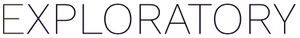# How to calculate 30 days standard deviation in annualized term

#1

To calculate 30 days standard deviation in the annualized term, you need to multiply daily standard deviation by the square root of a period like 253 days. This assumes there are 253 trading days in a given year. Int this example, the period is stored in count column.

``````roll_sd(adjusted, n = 30, fill = NA) * sqrt(count)
``````

For getting trading days for a year, you can group by the time series financial data by the year, then create a count column by `n()`.